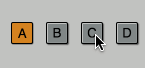Jamba Views / Discrete Button### Discrete ButtonA discrete button behaves like a toggle button except that it is `on` only if the control value (tied to a parameter) is equal to the `step` value (`DiscreteButtonView::getStep()` / `step` property in the xml). Selecting the button will set the underlying parameter to `step`. This button can be used to implement a radio group button behavior (only one selected at a time).

This view works for any parameter (both Vst and Jmb) that is (or can be interpreted as) a discrete parameter.

In addition to the attributes exposed by `CustomDiscreteControlView`, this class exposes the following attributes:

Attribute Description
`frames` The number of frames the image contains. Should be either 2 or 4 (4 includes the pressed state).
`on-color` When no image is provided, `back-color` is used for the “off” state and `on-color` for the “on” state (draws a rectangle with this color).
`button-image` The image for the button (2 or 4 frames).
`inverse` Inverses the meaning of “on” and “off” in regards to drawing the view/image.
`step` The value used to check whether the button is “on” or “off” as well as the value to set the parameter to when the button is selected.

Like the toggle button, it can have 2 or 4 frames (handle depressed state).

The image above shows an example of a vst parameter representing a bank (A through D) of pads. The vst parameter is backed by a discrete value (a VST parameter where step count > 0). The UI shows 4 discrete buttons each one tied to a different step. Because a discrete button is `on` only when the vst parameter matches its step, selecting one will automatically deselect the others.

#### Example

The XML defining the 4 buttons (Source)

``````<view class="jamba::DiscreteButton" control-tag="Param_PadBank" step="0" frames="2" origin="415, 296" size="26, 26" ... />
<view class="jamba::DiscreteButton" control-tag="Param_PadBank" step="1" frames="2" origin="450, 296" size="26, 26" ... />
<view class="jamba::DiscreteButton" control-tag="Param_PadBank" step="2" frames="2" origin="485, 296" size="26, 26" ... />
<view class="jamba::DiscreteButton" control-tag="Param_PadBank" step="3" frames="2" origin="520, 296" size="26, 26" ... />
``````

The vst param is defined this way (discrete parameter => represented by int) (Source)

``````VstParam<int> fPadBank;
``````

The vst param is initialized this way (Source)

``````fPadBank =